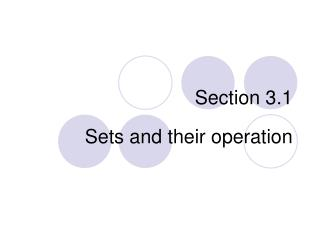Download PresentationSection 3.1

# Section 3.1

Download Presentation## Section 3.1

- - - - - - - - - - - - - - - - - - - - - - - - - - - E N D - - - - - - - - - - - - - - - - - - - - - - - - - - -
##### Presentation Transcript

1. Section 3.1 Sets and their operation

2. Definitions A set S is collection of objects. These objects are said to be members or elements of the set, and the shorthand for writing “x is an element of S” is “x S.” The easiest way to describe a set is by simply listing its elements (the “roster method”). For example, the collection of odd one-digit numbers could be written {1, 3, 5, 7, 9}. Note that this is the same as the set {9, 7, 5, 3, 1} since the order elements are listed does not matter in a set.

3. Examples The elements of a set do not have to be numbers as the following examples show: • {Doug, Amy, John, Jessica} • {TTT, TTF, TFT, TFF, FTT, FTF, FFT, FFF} • { {A,B}, {A,C}, {B,C} } • { }

4. Common sets of numbers Page 182 • N … set of natural numbers {0, 1, 2, …} • Z … set of integers {…, -2, -1, 0, 1, 2, …} • Q … set of rational numbers • R … set of real numbers

5. Definitions If A and B are sets, then the notation AB (read “A is a subset of B”) means that every element of set A is also an element of set B. Practice. Which is true? • {1, 2, 3, 4}  {2, 3, 4} • ZQ • ZN • { }  {a, b, c} • {3, 5, 7}  {2, 3, 5, 7, 11} • {a, b}  { {a, b}, {a, c}, {b, c} } • {a}  { {a, b}, {a, c}, {a, b, c} }

6. Set notation Large sets cannot be listed in this way so we need the more compact “set-builder” notation. This comes in two types exemplified by the following: • (Property) {nZ : n is divisible by 4} • (Form) {4k : kZ}

7. Practice with property description List five members of each of the following sets: • {nN : n is an even perfect square } • {xZ : x – 1 is divisible by 3 } • {rQ : r2 < 2 } • {xR : sin(x) = 0 }

8. Practice with form description List five members of each of the following sets: • { 3n2 : nZ } • { 4k + 1 : kN } • { 3 – 2r : rQ and 0 r 5 }

9. Definitions of set operations Let A and B be sets with elements from a specified universal set U. • AB (read “A intersect B”) is the set of elements in both sets Aand B. • A B (read “A union B”) is the set of elements in either set Aor B. • A – B (read “A minus B”) is the set of elements in set Awhich are not in B. • A’ (read “the complement of A”) is the set of elements in the universe Uwhich are not in A.

10. Practice with set operations Let A = {1, 3, 5, 7, 9}, B = {2, 4, 6, 8, 10}, C = {2, 3, 5, 7}, D = {6, 7, 8, 9, 10} be sets with elements from the universal set U = {1, 2, 3, 4, 5, 6, 7, 8, 9, 10} Find each of the following: • A  C • B  D • B – D • B’ • (A  B) – C • (A  C)  B • B’  C’ • (B  C)’ • (C  D) – A • B  D’

11. Venn diagrams

12. Inclusion-Exclusion Principle The notation n(A) means “the number of elements of A.” For example, if A = {2, 3, 6, 8, 9}, then n(A) = 5. Principle of Inclusion/Exclusion for two sets A and B: n(AB) = n(A) + n(B) – n(AB)

13. Inclusion-Exclusion Principle Example. A = { 2, 4, 6, 8, …, 96, 98, 100 } and B = { 5, 10, 15, 20, …, 90, 95, 100} n(AB) = n(A) + n(B) – n(AB) = 50 + 20 – 10 = 60

14. Inclusion-Exclusion Principle Principle of Inclusion/Exclusion for three sets A, B, and C: n(AB C) = n(A) + n(B) + n(C) – n(AB) – n(AC) – n(BC) + n(ABC)